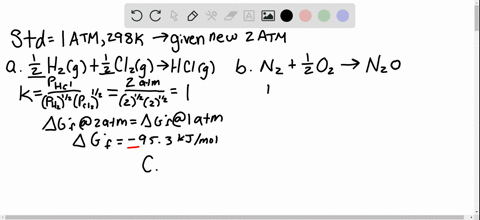Enroll in one of our FREE online STEM summer camps. Space is limited so join now!View Summer Courses### Suppose we redefine the standard state as $P=2$ a…

09:25
LP

Need more help? Fill out this quick form to get professional live tutoring.

Get live tutoring
Problem 104

Calculate the entropy of each state and rank the states in order of increasing entropy.
a.
b.
c.

PART A: $S=0 \mathrm{J} / \mathrm{K}$
PART $\mathrm{B} : S=3.18 \times 10^{-23} \mathrm{J} / \mathrm{K}$
PART $\mathrm{C} : S=2.22 \times 10^{-23} \mathrm{J} / \mathrm{K}$
$(\mathrm{a}) < (\mathrm{c}) < (\mathrm{b})$

## Discussion

You must be signed in to discuss.

## Video Transcript

So this question wants youto rank the three states in order of his increasing energy entropy and to also conquered the interview for each of the states. So to calculate, entropy used this equation S, which is inch B equals K natural log of W. Where Kay is the bolts been constant when she cools 1.38 times 10 to the minus 23 tend to manage 23 jewels For Calvin, it's a constant in your textbook now W's number of micro states critically, the unique ways you can range the adams for that givens macro state. So Kay's given to us a mutually zero W. Is, and we can cancan identity. So for a question, Part one, there's five. Adam's all in left side, and so the little you can arrange this is have all five Adams on the left side. There's no swapping of atoms that can occur that's unique. So the number micro states is equal to one. So entropy equals K natural log of one unnatural long of 10 So entropy is zero. So the next one is you have three on the left and two on the right and so few region textbook. Um, there's a section where they tell you a quick way to calculate W according to this, and it's and factorial over and minus R factorial times are effect world where and is the total number of Adams and our is the number of atoms on the left side or the right side. Either way, it's okay. It's over this case and equals five and r equals three. Just pick. So now W If you plug those numbers in, I'll give you w equals 10. So now, in completed, I am for S and then gets you 3.1 seven 756 approximately temps into the mice. 23 Jules Per Calvin says for the 2nd 1 the 3rd 1 see corner left and one on the right. And seven calculated using the same formula with an equaling five again. And now our can equal four. And this gives you w If you plug that in, you get the vehicles five into s equals. Que natural log of five, which he calls 2.22 times 10 to the minus 23. Approximately jewels for Calvin. And so now we can have our entry. We can rank these in quarter of an order of increasing entropy. So if you look back at the values, we have a being the lowest deceiving second and be being having the most entropy. So that's how you would drink the three.